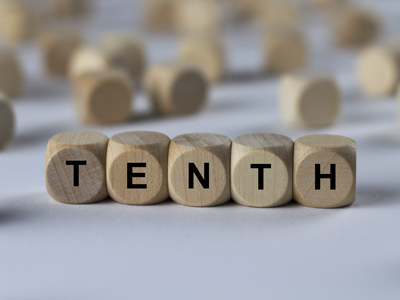The first number after a decimal point is in the tenths' place.

# Place Value 2 (Difficult)

Welcome to the second of our Difficult level Eleven plus maths quizzes on Place Value. By now you should be very familiar with the way the values of digits change depending on their position in a number. If not, then don’t worry – here’s another chance to practice with whole and decimal numbers.

If you’ve forgotten, here’s a quick reminder:

Place values BEFORE the decimal point are read RIGHT to LEFT with the following values – Ones, Tens, Hundreds, Thousands, Ten thousands.

Place values AFTER the decimal point are read LEFT to RIGHT with the following values – Tenths, Hundredths, Thousandths, Ten thousandths.

Make sure you read each question thoroughly – it’s very easy to mistake ‘hundreds’ for ‘hundredths’!

1.
What is the place value of 0 in 1,904.2693?
The tenths' place
The ones' place
The thousands' place
The tens' place
Reading the number from right to left: 0 is in the tens' place
2.
What is the digit in the tens' place in 1,924.86?
2
8
4
6
Reading the number from right to left: 2 is in the tens' place
3.
What is the digit in the ones' place in 2,340.19?
4
0
1
9
Reading the number from right to left: 0 is in the ones' place
4.
What is the place value of 1 in 90,462.315?
The hundreds' place
The tens' place
The hundredths' place
The tenths' place
Reading from left to right immediately after the decimal point: 1 is in the hundredths' place
5.
What is the place value of 6 in 21,796.025?
The ones' place
The tens' place
The tenths' place
The thousands' place
Reading the number from right to left: 6 is in the ones' place
6.
What is the digit in the ten thousands' place in 45,863.0201?
1
4
0
5
Reading the number from right to left: 4 is in the ten thousands' place
7.
What is the digit in the ten thousandths' place in 70,245.0908?
7
0
8
9
Reading from left to right immediately after the decimal point: 8 is in the ten thousandths' place
8.
What is the digit in the hundredths' place in 1,346.9782?
3
8
7
4
Reading from left to right immediately after the decimal point: 7 is in the hundredths' place
9.
What is the place value of 3 in 2.0136?
The hundredths' place
The thousands' place
The hundreds' place
The thousandths' place
Reading from left to right immediately after the decimal point: 3 is in the thousandths' place
10.
What is the place value of 4 in 40,728.0693?
The ten thousandths' place
The thousandths' place
The ten thousands' place
The thousands' place
Reading the number from right to left: 4 is in the ten thousands' place
Author:  Frank Evans